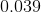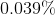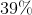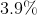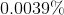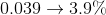## Example Questions

← Previous 1

### Example Question #1 : Decimals And Percentage

If 20% of x is equal to 160% of y, then y is what percent of x?

8

12.5

125

800

1.25

12.5

Explanation:

We are told that 20% of x is equal to 160% of y. We need to write expressions for 20% of x and 160% of y and set them equal to one another.

In order to find an expression for 20% of x, we can write 20% as a decimal and multiply it by x. Since 20% = 0.20, we can write 20% of x as 0.2x.

Similarly, we can write 160% as 1.60y.

Now, we set these expressions equal to one another.

0.2x = 1.60y.

Since the question asks us to find y as a percentage of x, we need to solve for y in terms of x. Let's divide both sides of the equation by 1.60.

0.125x = y.

Therfore, y is equal to 0.125x, which is the same as 12.5% of x, since 12.5% expressed as a decimal is 0.125.

### Example Question #65 : Percentage

Find the percentage equivalent of the decimal: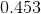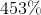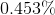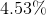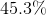Explanation:

In order to find the percentage equivalent of a decimal, the decimal has to be multiplied by 100. However, since it is multiplication by a power of 10, we can accomplish the same thing, by moving the decimal point 2 places to the right, thus making the number larger. For this problem, that looks like this: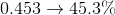### Example Question #1 : How To Find Percentage Equivalent To A Decimal

Find the percentage equivalent of the decimal: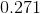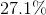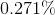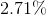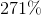Explanation:

In order to find the percentage equivalent of a decimal, the decimal has to be multiplied by 100. However, since it is multiplication by a power of 10, we can accomplish the same thing, by moving the decimal point 2 places to the right, thus making the number larger. For this problem, that looks like this: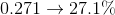### Example Question #67 : Percentage

Find the percentage equivalent of the decimal: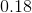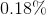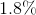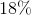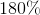Explanation:

In order to find the percentage equivalent of a decimal, the decimal has to be multiplied by 100. However, since it is multiplication by a power of 10, we can accomplish the same thing, by moving the decimal point 2 places to the right, thus making the number larger. For this problem, that looks like this: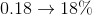### Example Question #68 : Percentage

Find the percentage equivalent of the decimal: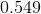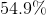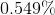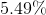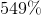Explanation:

In order to find the percentage equivalent of a decimal, the decimal has to be multiplied by 100. However, since it is multiplication by a power of 10, we can accomplish the same thing, by moving the decimal point 2 places to the right, thus making the number larger. For this problem, that looks like this: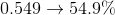### Example Question #69 : Percentage

Find the percentage equivalent of the decimal: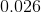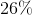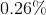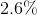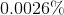Explanation:

In order to find the percentage equivalent of a decimal, the decimal has to be multiplied by 100. However, since it is multiplication by a power of 10, we can accomplish the same thing, by moving the decimal point 2 places to the right, thus making the number larger. For this problem, that looks like this: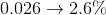### Example Question #70 : Percentage

Find the percentage equivalent of the decimal: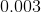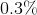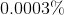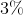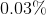Explanation:

In order to find the percentage equivalent of a decimal, the decimal has to be multiplied by 100. However, since it is multiplication by a power of 10, we can accomplish the same thing, by moving the decimal point 2 places to the right, thus making the number larger. For this problem, that looks like this: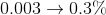### Example Question #21 : Decimals And Percentage

Find the percentage equivalent of the decimal: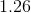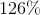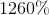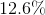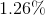Explanation:

In order to find the percentage equivalent of a decimal, the decimal has to be multiplied by 100. However, since it is multiplication by a power of 10, we can accomplish the same thing, by moving the decimal point 2 places to the right, thus making the number larger. For this problem, that looks like this: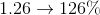### Example Question #22 : Decimals And Percentage

Find the percentage equivalent of the decimal: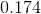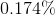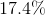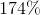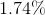Explanation:

In order to find the percentage equivalent of a decimal, the decimal has to be multiplied by 100. However, since it is multiplication by a power of 10, we can accomplish the same thing, by moving the decimal point 2 places to the right, thus making the number larger. For this problem, that looks like this: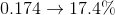### Example Question #23 : Decimals And Percentage

Find the percentage equivalent of the decimal: